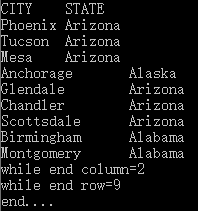2020-02-17 15:52:50

# ODBC 服务

## 启动 ODBC 服务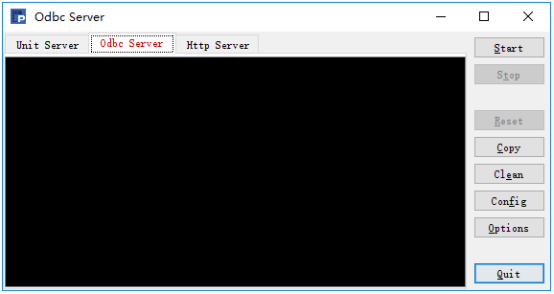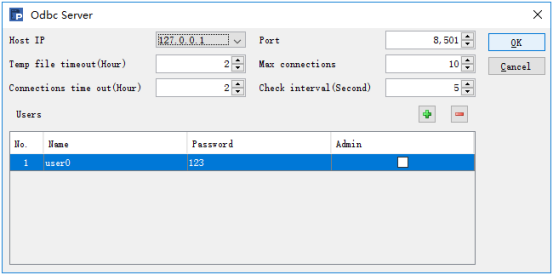ODBC 服务配置中主要配置 IP、ODBC 服务的端口、允许访问的用户名和密码。配置完成后，点击【OK】，保存配置。然后点击【start】启动服务。

## 添加 ODBC 数据源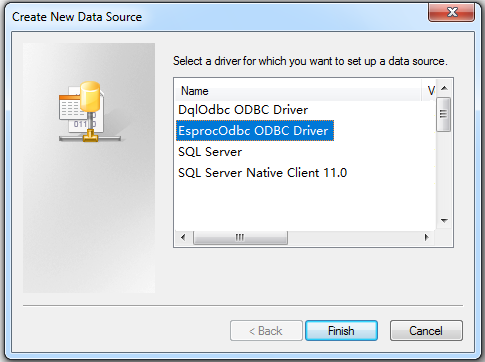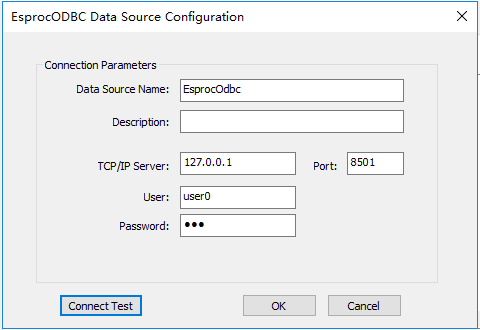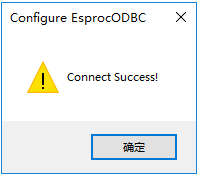# C# 调用

## 执行 SPL 语句

C# 代码如下：

```using System;

using System.Collections.Generic;

using System.Linq;

using System.ComponentModel;

using System.Text;

using System.Data.Odbc;

namespace ODBCtest

{

classDB

{

publicvoid rset(OdbcConnectionconn, string selectSql)

{

OdbcCommandcmd = newOdbcCommand(selectSql, conn);

int nCount = 0;

//循环输出列名

{

}

Console.Write("\\n");

{

nCount++;

}

Console.WriteLine("while end row=" \+ nCount);

}

}

classProgram

{

staticvoid Main(string\[\] args)

{

//生成ODBC连接字符串,其中DSN、UID、PWD属性依次表示ODBC数据源名称、用户名、密码

stringconstr = "DSN=EsprocOdbc;"\+ "UID=user0;"\+ "PWD=123;";

OdbcConnectionconn = newOdbcConnection(constr);

conn.Open();

//要执行的SPL语句

stringspl = "10.new(~:baseNum,~*~:square2)";

DBdb = newDB();

db.rset(conn, spl);

Console.Write("end....");

conn.Close();

}

}

}

using System;

using System.Collections.Generic;

using System.Linq;

using System.ComponentModel;

using System.Text;

using System.Data.Odbc;

namespace ODBCtest

{

classDB

{ publicvoid rset(OdbcConnectionconn, string selectSql)         {

OdbcCommandcmd = newOdbcCommand(selectSql, conn);

{

}

{

nCount++;

}

Console.WriteLine("while end row=" \+ nCount);

}

}

classProgram

{ staticvoid Main(string\[\] args)         { //生成ODBC连接字符串,其中DSN、UID、PWD属性依次表示ODBC数据源名称、用户名、密码 stringconstr = "DSN=EsprocOdbc;"\+ "UID=user0;"\+ "PWD=123;";

OdbcConnectionconn = newOdbcConnection(constr);

conn.Open(); //要执行的SPL语句 stringspl = "10.new(~:baseNum,~*~:square2)";

DBdb = newDB();

db.rset(conn, spl);

Console.Write("end....");

conn.Close();

}

}

} ```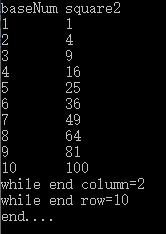## 在 SPL 中访问本地文件

```

D:\\mainFile

<mainPath>D:\\mainFilemainPath> ```

```stringspl = "=file(\\"employee.txt\\").import@t()";//SPL语句

stringspl = "=file(\\"employee.txt\\").import@t()";//SPL语句 ```

```while (reader.Read())

{

nCount++;

}

{

nCount++;

} ``````stringspl = "\$select * from employee.txt";

stringspl = "\$select * from employee.txt"; ```

## 带参数的 SPL 语句

```stringspl = "\$select *
from employee.txt
where SALARY > ? and SALARY< ?
order by SALARY";

stringspl = "\$select *
from employee.txt
where SALARY > ? and SALARY< ?
order by SALARY"; ```

rset 方法中的传参部分代码如下:

```OdbcCommandcmd = newOdbcCommand(selectSql, conn);

OdbcCommandcmd = newOdbcCommand(selectSql, conn);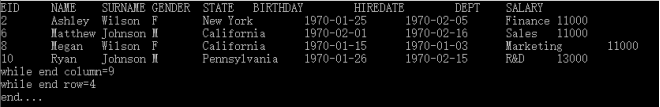## 有数据源的 SPL 语句

C# 调用带数据源的 SPL 语句之前，需要先在应用项目中添加对应的数据库驱动，然后在配置文件 raqsoftConfig.xml 中配置数据源信息。

```

<DBList> <DB name="dm">  <property name="url" value="jdbc:hsqldb:hsql://127.0.0.1/demo" />  <!\-\- url连接--> <property name="driver" value="org.hsqldb.jdbcDriver" />   <property name="type" value="13" />  <property name="user" value="sa" />   <property name="password" value=""/>   <property name="batchSize" value="1000" /> <property name="autoConnect" value="true" />  <property name="useSchema" value="false" /> <property name="addTilde" value="false" /> <property name="dbCharset" value="UTF-8" /> <property name="clientCharset" value="UTF-8" /> <property name="needTransContent" value="false" /> <property name="needTransSentence" value="false" /> <property name="caseSentence" value="false" /> DB> DBList> ```

```stringspl = "\$(dm)select *
from SALES
where  SELLERID = ? and  ORDERDATE>TO\_DATE(?,'YYYY-MM-DD') and  ORDERDATE

```
stringspl = "\$(dm)select * from SALES   where  SELLERID = ? and  ORDERDATE>TO\_DATE(?,'YYYY-MM-DD') and  ORDERDATE;

rset 方法中参数传递部分代码如下：

``` cmd.Parameters.Add("arg1", OdbcType.Int).Value = 3;

```while (reader.Read())

{

nCount++;

}

{

nCount++;

} ```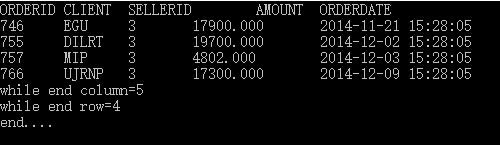## 执行 SPL 脚本

C# 调用 ODBC，除了可以直接执行单句的 SPL 语句，还可以调用更复杂的 SPL 脚本（后缀为 dfx 的文件）。

 A B C 1 =connect("dm").query("select NAME as CITY, STATEID as STATE from   CITIES") [] 2 for A1 =dm.query("select * from STATES where   STATEID=?",A2.STATE) 3 if left(B2.ABBR,1)==arg1 >A2.STATE=B2.NAME 4 >B1=B1|A2 5 return B1

SPL 脚本思路：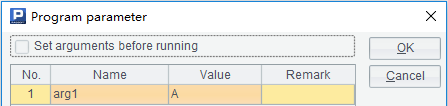```

D:\\dfxFile

<dfxPathList>       <dfxPath>D:\\dfxFiledfxPath>dfxPathList> ```

```stringspl = "call city(?)";

stringspl = "call city(?)"; ```

rset 方法中传参部分代码如下：

```  cmd.Parameters.Add("arg1", OdbcType.VarChar).Value = "A";

``` while (reader.Read())

{

nCount++;

}

{

nCount++;

} ```

```stringspl = "call city('A')";

stringspl = "call city('A')"; ```